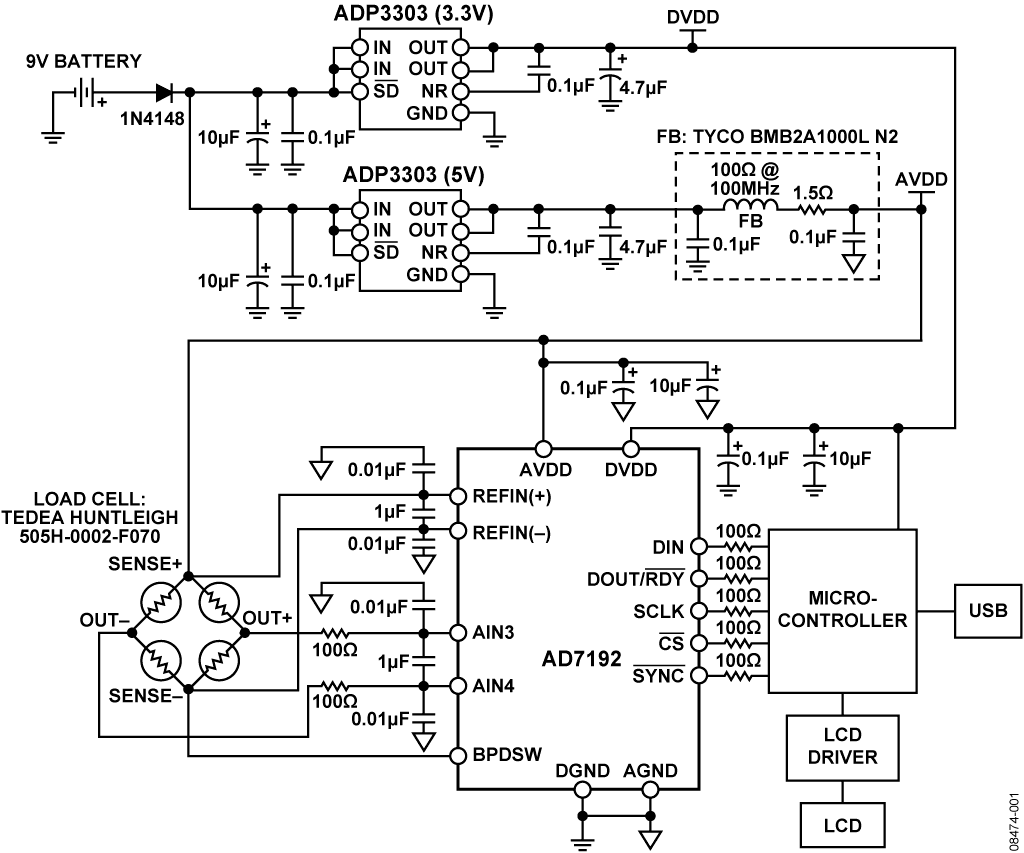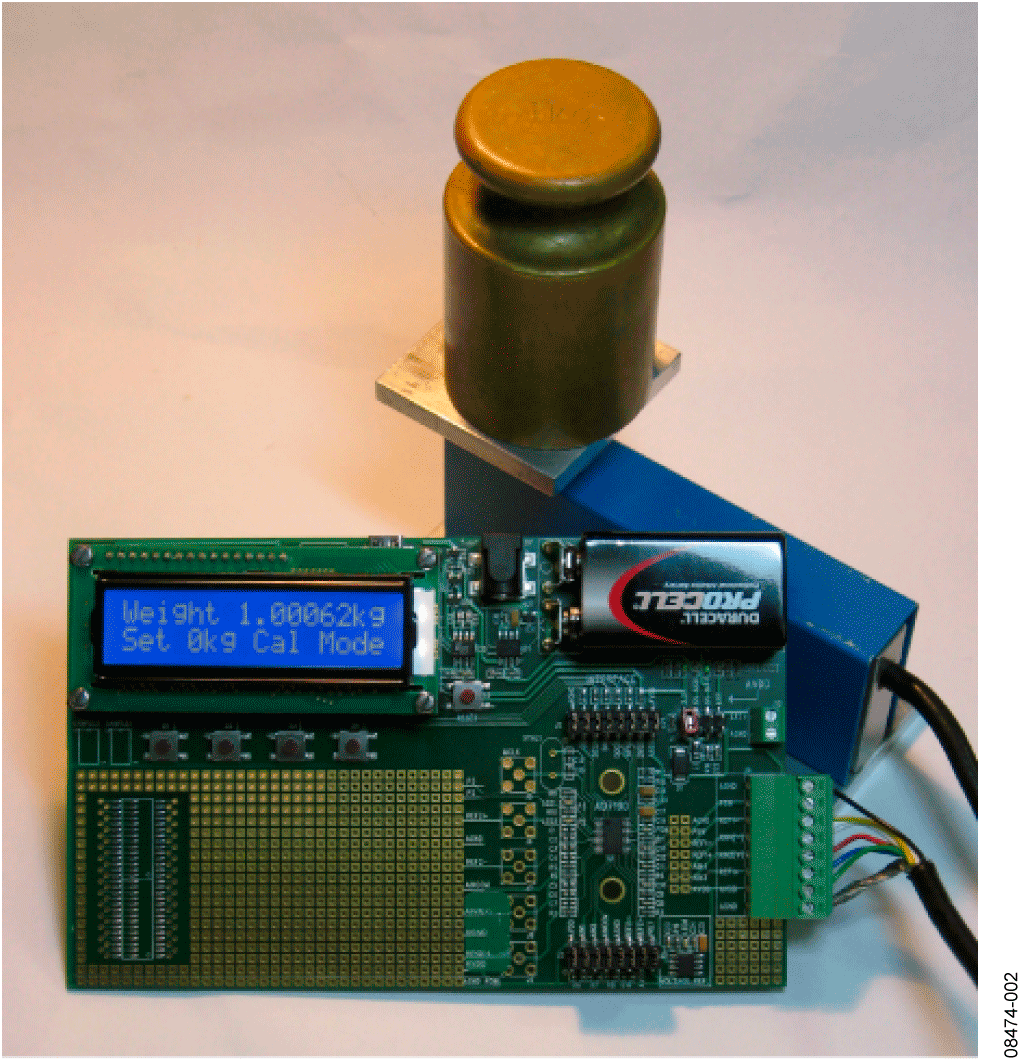# 概要

## 設計リソース

### デバイス・ドライバ

Software such as C code and/or FPGA code, used to communicate with component's digital interface.

## 機能と利点

• Handles fast data rates up to 4.8 kHz
• 24-bit pressure application circuit
• Integrated PGA allows for wide array of sensors

# 回路機能とその特長

This circuit is a weigh scale system that uses the AD7192. The AD7192 is an ultralow noise, low drift, 24-bit Σ-Δ converter that includes a PGA. Therefore, the device simplifies the weigh scale design because most of the system building blocks are included on chip. Since the part operates with an output data rate from 4.7 Hz to 4.8 kHz and maintains good performance over the complete output data rate range, this allows the part to be used in weigh scale systems that operate at low speeds along with higher speed weigh scale systems, such as hopper scales.Figure 1. Weigh Scale System Using the AD7192 (Simplified Schematic: All Connections Not Shown)

# 回路説明

Since the AD7192 provides an integrated solution for weigh scales, it interfaces directly to the load cell. The only external components required are some filters on the analog inputs and capacitors on the reference pins for EMC purposes. The low level signal from the load cell is amplified by the AD7192's PGA. The PGA is programmed to operate with a gain of 128. The conversions from the AD7192 are then sent to the microcontroller where the digital information is converted to weight and displayed on the LCD.Figure 2. Weigh Scale System Using the AD7192

The AD7192 has separate analog and digital power supplies. The digital power supply is independent of the analog power supply and can equal any voltage between 2.7 V and 5.25 V. The micro-controller uses a 3.3 V power supply. Therefore, DVDD is also powered from 3.3 V. This simplifies the interface between the ADC and the microcontroller because no external level shifting is required.

The AD7192 has an rms noise of 11 nV when the output data rate is 4.7 Hz. The number of noise-free counts is equal towhere the factor of 6.6 converts the rms voltage into a peak-to-peak voltage.

The resolution in grams isThe noise-free code resolution is equal toIn practice, the load cell itself will introduce some noise. There will also will be some drift due to time and temperature of the load cell along with the AD7192's drift. To determine the accuracy of the complete system, the weigh scale can be connected to the PC via the USB connector. Using LabView software, the performance of the weigh scale system can be evaluated. Figure 4 shows the measured output performance when a 1 kg weight is placed on the load cell and 500 conversions are gathered. The noise of the system is calculated by the software to be 14 nV and is 98 nV peak-to-peak. This equates to 102,000 noise-free counts or 16.6 bits of noise-free code resolution.Figure 3. Measured Output Code for 500 Samples Showing the Effects of NoiseFigure 4. Measured Output Code for 500 Samples Showing the Effects of Noise

Figure 4 shows the performance in terms of weight. The variation in output is 0.02 grams over the 500 codes. So, the weigh scale system achieves an accuracy of 0.02 grams.

The plots show the actual conversions being read back from the AD7192 when the load cell is attached. In practice, a digital post filter is used in a weigh scale system. The additional averaging that is performed in the post filter will further improve the number of noise-free counts at the expense of a reduced data rate.

# バリエーション回路

Note: All noise specifications in this section are given for a PGA gain of 128.